# Self driving cars: From A to Z(Module 1) — 1

Source: Deep Learning on Medium

Welcome to module 1- part 1 of Self driving car engineer. This part deals with computer vision fundamentals which will help us in finding lane lines in images using computer vision techniques. So lets get started.

Suppose we want to find lane lines in the image below.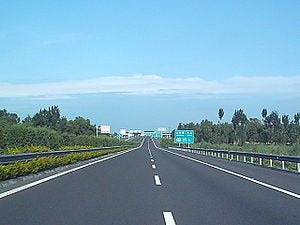pic courtesy: en.wikipedia.org

A usual color image is made up of 3 channels namely Red, Green and Blue with values ranging from 0 to 255(0 being black and 255 white) as displayed below:

When a color image is imported to python it is saved as a multidimensional array/Tensor with the dimensions being height, width and depth respectively.

`import matplotlib.pyplot as pltimport matplotlib.image as mpimgimport numpy as np# Read in the imageimage = mpimg.imread('test.jpg')# Grab the x and y size and make a copy of the imageysize = image.shapexsize = image.shapered_threshold = 200green_threshold = 200blue_threshold = 200rgb_threshold = [red_threshold, green_threshold, blue_threshold]`

Now if we have to detect lanes from the above image a naive approach would be to set a color threshold for each of the RGB channel. As mentioned, these channels hold values from 0 to 255 with 255 being white, we can directly set a threshold for each of the channel somewhere close to 200 or above and try to see if lanes are detected as expected. When I say setting a threshold it means that any value that is below the threshold will be set to zero and only values above the threshold will be seen in the image. After applying the threshold selection method the below image was generated.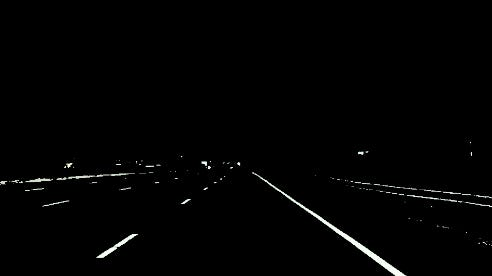threshold value : 200

The threshold selection method did a pretty decent job with eliminating most of the unwanted regions in the image. But there still exists some other objects in the boundary that are not actually lanes.

Usually Self driving cars have cameras located in fixed positions which can be either at the top of the car or on the front. In both the cases the lane lines will be appearing in the same general region of the image. We can use this logic to our advantage by only considering the pixels in the region of the image where we expect to find the car.

Now we know the region of interest(ROI) in the image, so we can define a triangle region of interest and fit a model for line for each of the sides of the triangle. We need to keep in mind that the origin(x=0, y=0) is in the upper left in image processing. We need to define left_bottom, right_bottom and apex such that we cover the ROI and get the threshold for our region of interest. I tried with various combinations for left_bottom, right_bottom and apex to get the right set of combination. After getting the right combination we can perform a linear fit for each side of a triangle using np.polyfit() functions which returns the slope and intercept for each of the line which are the sides of the triangle.

`left_bottom = [120, 539]right_bottom = [800, 539]apex = [470, 300]# Perform a linear fit (y=Ax+B) to each of the three sides of the triangle# np.polyfit returns the coefficients [A, B] of the fitfit_left = np.polyfit((left_bottom, apex), (left_bottom, apex), 1)fit_right = np.polyfit((right_bottom, apex), (right_bottom, apex), 1)fit_bottom = np.polyfit((left_bottom, right_bottom), (left_bottom, right_bottom), 1)`

After performing a linear fit on each of the side of the triangle we can now define the threshold for ROI and any value that is below the threshold is set to 0 as before.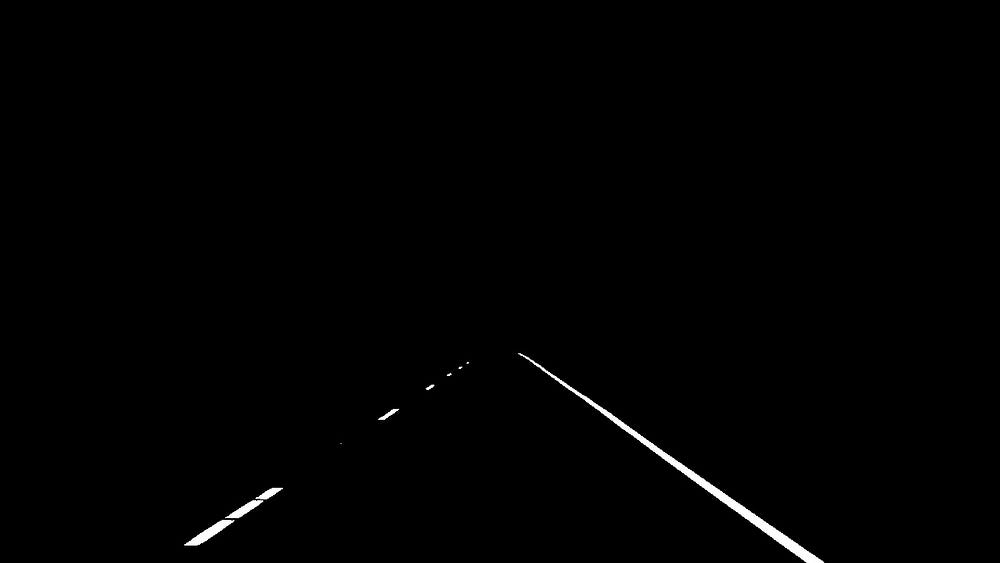After applying linear fit and threshold selection

So we can see that we are able to detect lane lines accurately using this method. Do you think we can upload this code into our self-driving car and get going?? Not yet. The lane lines will not always be of same color, and color might appear differently in different light conditions e.g. day or night. In such cases our algorithm might fail to detect lanes by just applying color selection method. We need to build an algorithm that can detect lane lines in any color and under any lighting conditions. Thus we need to apply more advanced computer vision techniques which would solve our purpose.

We will be using canny edge detection technique which comes built-in in opencv package. The goal of edge detection is to identify the boundaries of an object in an image. In order to perform edge detection first the image is converted to gray scale and then compute the gradient. The brightness of each pixel corresponds to the strength of the gradient at that point. we then find the edges by tracing out the pixels that follow strongest gradients. The canny function in opencv looks like this:

edges = cv2.Canny(gray, low_threshold, high_threshold)

It takes in a grayscale image and has two more parameters to be specified: low_threshold and high_threshold. These threshold defines how strong the edges must be to be detected. When we look at a gray scale image we can see bright and dark pixels and all the shades of it. A sudden change in pixel values is where the edges are detected

An image is just like a matrix in the back end where we can apply any function as we do with any other matrices. The function will be applicable to the pixel values of the image. For e.g. we can take derivative of df/dx which implies change in pixel values. A small derivative implies small change and a big derivative implies big change in the pixel values. Computing gradients of the image gives us thick edges, but we can use the canny function to thin out these edges. The following image is generated after applying the canny function with low_threshold of 50 and high_threshold of 150. You are free to play with these values to get more accurate images.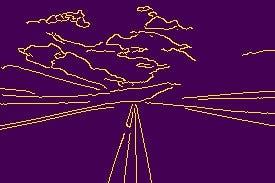Canny generated image(low_threshold:50, high_threshold:150)

Now lets recap, we took a colored image converted it to gray scale and using canny edge detection computed and gradients and generated an image full of dots. But we would require dots that represents the lane lines in the original image. In order to identify lines we need to adopt model of a line and then fit that model to the dots in the edge detected image.As we know that an image is just a function of x and y we can use the trivial equation of a line which is:

y = mx +b

In this case we have two parameters m and b. In image space a line is represented as y = m0x + b0 but in parameter space or hough space it is represented as a point as shown below.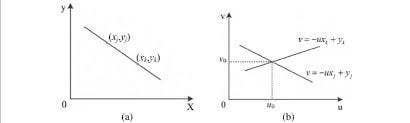Image space(left) , Hough Space(right)

New we apply hough transformation to find the lane lines in our image. There are some parameters that needs to be specified before applying the transformation. Description for each is given in the code below:

`# Define the Hough transform parameters# Make a blank the same size as our image to draw onrho = 1 # distance resolution in pixels of the Hough gridtheta = np.pi/180 # angular resolution in radians of the Hough gridthreshold = 2     # minimum number of votes (intersections in Hough grid cell)min_line_length = 4 #minimum number of pixels making up a linemax_line_gap = 5    # maximum gap in pixels between connectable line segmentsline_image = np.copy(image)*0 # creating a blank to draw lines on# Run Hough on edge detected image# Output "lines" is an array containing endpoints of detected line segmentslines = cv2.HoughLinesP(masked_edges, rho, theta, threshold, np.array([]),                            min_line_length, max_line_gap)# Iterate over the output "lines" and draw lines on a blank imagefor line in lines:    for x1,y1,x2,y2 in line:        cv2.line(line_image,(x1,y1),(x2,y2),(255,0,0),10)`

We can select the ROI using the triangle method above or we can replace triangle with polynomial to get better results something like this:

vertices = np.array([[(0,imshape),(450, 290), (490, 290), (imshape,imshape)]], dtype=np.int32)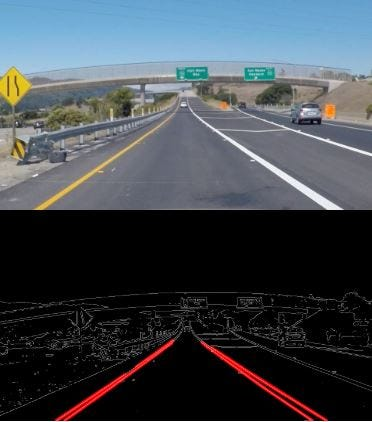Original image(up), final image after transformations(below)

Finally we come to the end of part 1 of the first module. It was a good experience conveying what I learnt from this Self driving car Nano degree. I hope I was able to help you guys understand the basics of computer vision and lane finding techniques using opencv. You can comment on how did you like the post and how you guys want me to address the future blogs of this series.

You can find the code to this section at https://github.com/ChandanVerma/SelfDrivingCar/tree/master/Module%2001-Computer%20Vision%20Fundamentals

References:

academictorrents.com

www.researchgate.net

https://www.google.co.in/imghp?hl=en&tab=wi&authuser=0